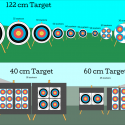# Archery Target Distance-Angle Equivalencies

One question that comes up from time to time is “How hard is it to hit a target [some distance] away?”.  The answer is a firm and definite “it depends.”

There are a variety of target sizes, shot at a variety of distances.  Even ignoring things like wind, air friction, body-angle to reach distances, etc, the size that the target appears to your eye changes a lot just due to distance.

Coming up to a 90 meter fun shoot, I was curious what my 70 meter score would roughly translate to at the further distance.  So I wrote up a Google spreadsheet to compute the difference in the target size.

As a warning, there’s some math involved.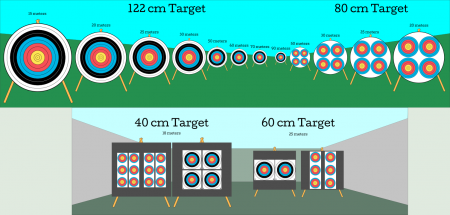# The Math

Ok, so remember when you asked your math teacher “When will I ever use this in real life?”  Today’s that day!  We’re going to calculate the apparent angle of the target at various distances.  The Apparent Angle is the angle that appears in your eyes.  That’s different at each distance, and for each size of target.  But since we have standard targets and standard distances, the apparent angles of each combination will be the same for every archer.

So, we draw some triangles to frame the distance to the target, and the diameter of the target circle: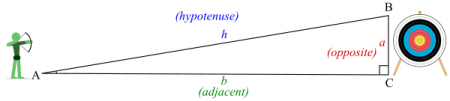The apparent angle will be angle ‘A’ in this diagram.  Since the distance to the target is ‘b – adjacent’ and the diameter of the target is ‘a – opposite’, then we’ll want to use the trigonometric function tangent.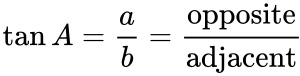Since the tangent(angle A) is the same ratio as opposite / adjacent, we can use that in reverse to find the angle A.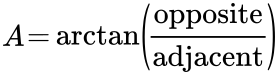So we take the target diameter, divide it by the distance to the target, then dump that into a the arctan (or tan-1 function).  That gives us the angle.  (Google Sheets does all of its trig in radians, so we use the radians or degrees functions to convert to the units we want to display.)

By computing the apparent angle of various targets at different distances, I scaled some target diagrams to show their different relative sizes.

Once we know the angle of the whole target, we can use the target specifications from World Archery to determine the apparent angle for individual rings.  The linked spreadsheet does this hundreds of times to get its results.

The additional nifty result is that you can compare angles and equivalent diameters at different distances.  So if a 122cm target is a certain size at 70 meters, you can see what diameter at a closer distance is the same apparent angle, and practice your aiming with that shorter distance and smaller circle.

# The Results

For me, the answer to my question (“How well do I need to do at 70 meters to stay on the target at 90 meters?”) is this table right here:  I need to stay in the inner half of the 3 ring at 70 meters to stay on the 90 meter 122cm target.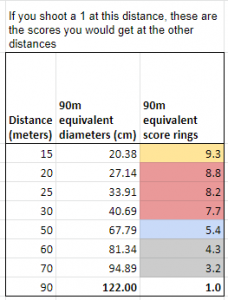At 70 meters, a diameter of about 95cm makes the same angle as 122cm at 90 meters.  The ~95cm falls inside the 3 ring, but not quite to the 4 ring.  If you’re practicing at 30 meters, you should probably stay inside the 8 ring.  These tables are all over in the spreadsheet.

For instance, a 122cm target (the big cheese wheels) at 70 meters is nearly exactly 1 degree of angle (I’m sure this was decided on purpose.)  That’s the same as the 9 ring on the 122cm target at 15 meters.  So if you’re practicing at 15 meters, and you can get all of your arrows into the 9 and 10 rings, you can aim well enough to hit the full 70 meter target! (ignoring some other factors — but your aim is great!).  But it you’re using all of the rings at 70 meters, some of those arrows will be misses at 90 meters, falling into the green ring — the grass…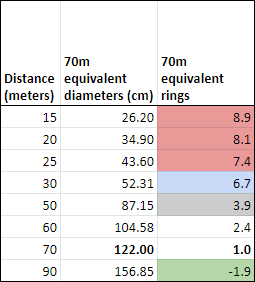And, at DT’s request, I added the indoor targets, as well.  Most importantly, here are the two numbers that show why during the indoor state championship your 25 meter score should be slightly higher than your 18 meter score — the apparent angle for the 25 meter round is higher, even with the longer distance.At the bottom of the spreadsheet is a section that tries to answer the question “How big is the bullseye from here?”.  That’s hard to answer.  But this uses the same arctan math from above to try to come up with some objects you can hold at arm’s length to be about the same size as the target or the gold.  For a lot of people, their index fingernail is about the size of the 122cm target at 70 meters.  Feel free to suggest things that would be good additions to this list.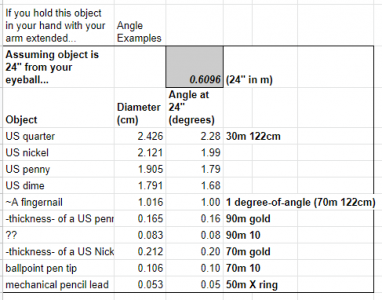### 4 Responses to Archery Target Distance-Angle Equivalencies

1.John says:

Awesome, exactly what I was looking for. Good article.

2.Stephen Collins says:

This is great! Any chance you could drop in 10m shooting distance? In these coronavirus times, I’m stuck shooting from my driveway into my garage and 10m is the furthest safe distance I can manage.

•Tony says:

I chucked in some 10m math. It’s been long enough since I’ve looked at that math that I’m blindly trusting me-from-the-past to have written formulae flexibly enough to give the right results.

•Stephen Collins says:

Awesome! Very much appreciated.